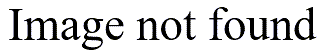# Confidence intervals for proportions

## 2004-07-27

One of the fellows at the hospital asked me about confidence intervals for proportions. I wrote a couple of simple spreadsheets to do these calculations

It’s important to avoid comparing two separate confidence intervals to see if they overlap. Someone brought me data where the proportion of patients who tested positive was 41.6% (n=202) for the first group and 50.7% (n=802) in the second group. The individual confidence intervals are (34.8% to 48.4%) and (47.2% to 54.2%). Notice that the two intervals overlap, but just barely. The confidence interval for the difference in two proportions, however, is (-16.7% to -1.5%) which provides evidence that the two proportions differ. This is a borderline result, of course, since one side of the interval almost reaches zero.

The reason that you can have overlap in the individual intervals is that you don’t add the two standard errors together. The standard error for the two individual intervals would beand the standard error for the difference isI also have spreadsheets that calculate confidence intervals for means:

These are not very sophisticated spreadsheets and they use the simplest formulas available. The nice thing, though, about these spreadsheets is that they allow you to play a bunch of “what if” games.

Exact confidence intervals

Some alternate confidence intervals based on the exact binomial distribution will provide better results than my spreadsheet, which uses the normal approximation to the binomial distribution. You can get such an interval using StatXact software, produced by Cytel, Inc. A paper (PDF format) at the their web site discusses some of these exact procedures and how to get p-values from an exact confidence interval.

Other pages that compute confidence intervals

There are lots of web pages out there that do confidence interval calculations, using Java or JavaScript. Here are a few nice examples of confidence intervals for a single proportion:

Exact Binomial and Poisson Confidence Intervals, John C. Pezzullo. members.aol.com/johnp71/confint.html

The Confidence Interval of a Proportion, Richard Lowry. faculty.vassar.edu/lowry/prop1.html

Large Sample Confidence Interval for a Proportion Applet, James W. Hardin. stat.tamu.edu/~jhardin/applets/signed/case6.html

and for the difference between two proportions:

The Confidence Interval for the Difference Between Two Independent Proportions, Richard Lowry. faculty.vassar.edu/lowry/prop2_ind.html

A nice general reference for web pages that do statistical calculations is

Web Pages that Perform Statistical Calculations. Pezzullo JC. Accessed on 2004-07-08. members.aol.com/johnp71/javastat.html

You can find an earlier version of this page on my original website.